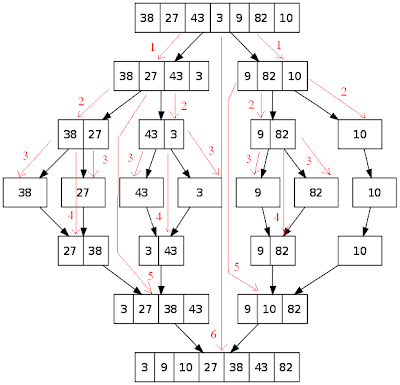## Wednesday, September 29, 2010

### What?

In my previous post about Merge Sort, the algorithm for merge sort was discussed. Although the pictures shows something which appears to be apparently parallel, but actually they are not. In fact, as the procedure introduced there was recursive, it works in a post-order fashion, meaning, first it solves the left half, then the right half, and then it works with the current range. The following picture shows the actual work-flow of the standard recursive merge sort algorithm:The red lines and numbers shows the actual call sequence of the algorithm merge sort, for reference, the basic algorithm, is shown here again:
`MERGE-SORT(A, p, r):    if p < r        then q := (r + p) / 2        MERGE-SORT(A, p, q)        MERGE-SORT(A, q+1, r)        MERGE(A, p, q, r)`

So, from the above example, we can see that no two calls are concurrent, i.e. parallel. Each call is one of the three calls made in MERGE-SORT(A, p, r) procedure stated above, also shown below (according to the picture, p=0, r=6):
`Step-00: MERGE-SORT(A, 0, 6)Step-01: MERGE-SORT(A, 0, 3)Step-02: MERGE-SORT(A, 0, 1)Step-03: MERGE-SORT(A, 0, 0)Step-04: MERGE-SORT(A, 1, 1)Step-05: MERGE(A, 0, 0, 1)Step-06: MERGE-SORT(A, 2, 3)Step-07: MERGE-SORT(A, 2, 2)Step-08: MERGE-SORT(A, 3, 3)Step-09: MERGE(A, 2, 2, 3)Step-10: MERGE(A, 0, 1, 3)Step-11: MERGE-SORT(A, 4, 6)Step-12: MERGE-SORT(A, 4, 5)Step-13: MERGE-SORT(A, 4, 4)Step-14: MERGE-SORT(A, 5, 5)Step-15: MERGE(A, 4, 4, 5)Step-16: MERGE-SORT(A, 6, 6)Step-17: MERGE(A, 4, 5, 6)Step-18: MERGE(A, 0, 3, 6)`

Also note that, there are no overlapping calls at a same level of the tree, which is going to be a key factor.

After a few observations, we can easily find out some interesting properties about merge sort algorithm. If we closely look at the merge sort tree structure, it becomes very clear that, int the recursive call tree, parent nodes depend on children, but siblings are independent. This property helps us to deduce a threaded version of merge sort algorithm where, we can really solve the left and right sub-portion of a range in a parallel fashion by making the calls threaded.

As the algorithm here is recursive, it might look confusing at the first glance, but it is not. Because, nodes share data only with their ancestors and in a call, we can wait until the threads finish their works. So, no concurrency problem appears here. Also, we don't need to worry about mutual exclusion of the shared data, because, there is no such situation in this algorithm, already stated above.

So, the threaded version of the algorithm will look like this:
`THREADED-MERGE-SORT(A, p, r):    if p < r        then q := (r + p) / 2        start_thread(left_thread, THREADED-MERGE-SORT(A, p, q))        start_thread(right_thread, THREADED-MERGE-SORT(A, q+1, r))        wait_for(left_thread)        wait_for(right_thread)        MERGE(A, p, q, r)`

According to the above algorithm, the flow of program changes which is shown in the picture below:Now because of being able to run multiple THREADED-MERGE-SORT() at a time, and the job scheduling features of modern computers, the effective number of iteration is dramatically reduced, resulting a much much elegant solution for the heavy duty areas like manipulating large data on drives.

### A simple implementation:

Here a simple implementation is presented using pthread library in C++:
`#include <stdio.h>#include <stdlib.h>#include <pthread.h>typedef struct { int i, j; } pair;const int MAX = 1024;int a[MAX];pthread_attr_t attr;void *threaded_merge_sort(void *param) {    pair range = *(pair *)param, lrange, rrange;    pthread_t lside, rside;    int p = range.i, q, r = range.j, n1, n2, n = r-p+1, i, j, k;    int *aleft, *aright;    if(p < r) {        q = (p + r) >> 1;        lrange.i = p, lrange.j = q, rrange.i = q + 1, rrange.j = r;        pthread_create(&lside, &attr, threaded_merge_sort, (void *)&lrange);        pthread_create(&rside, &attr, threaded_merge_sort, (void *)&rrange);        pthread_join(lside, NULL);        pthread_join(rside, NULL);        n1 = q - p + 1, n2 = r - q;        aleft = (int *)malloc(sizeof(int) * n1);        aright = (int *)malloc(sizeof(int) * n2);        for(i = 0; i < n1; i++) aleft[i] = a[p+i];        for(i = 0; i < n2; i++) aright[i] = a[q+1+i];        for(k = i = j = 0; k < n; k++) {            if(i >= n1 || (j < n2 && aleft[i] > aright[j])) a[k+p] = aright[j++];            else a[k+p] = aleft[i++];        }        free(aleft);        free(aright);    }    return NULL;}int main() {    int n, i;    pthread_t sorter;    pair range;    pthread_attr_init(&attr);    pthread_attr_setdetachstate(&attr, PTHREAD_CREATE_JOINABLE);    while(scanf("%d", &n)==1 && n) {        for(i = 0; i < n; i++) scanf("%d", &a[i]);        range.i = 0, range.j = n-1;        pthread_create(&sorter, &attr, threaded_merge_sort, (void *)&range);        pthread_join(sorter, NULL);        for(i = 0; i < n; i++) printf("%d%c", a[i], (i==n-1? '\n' : ' '));    }    pthread_attr_destroy(&attr);    return 0;}`

This may look a bit messy, but, actually, you may find a little difference with the original one, we just created threads instead of plain recursive calls, that's it.